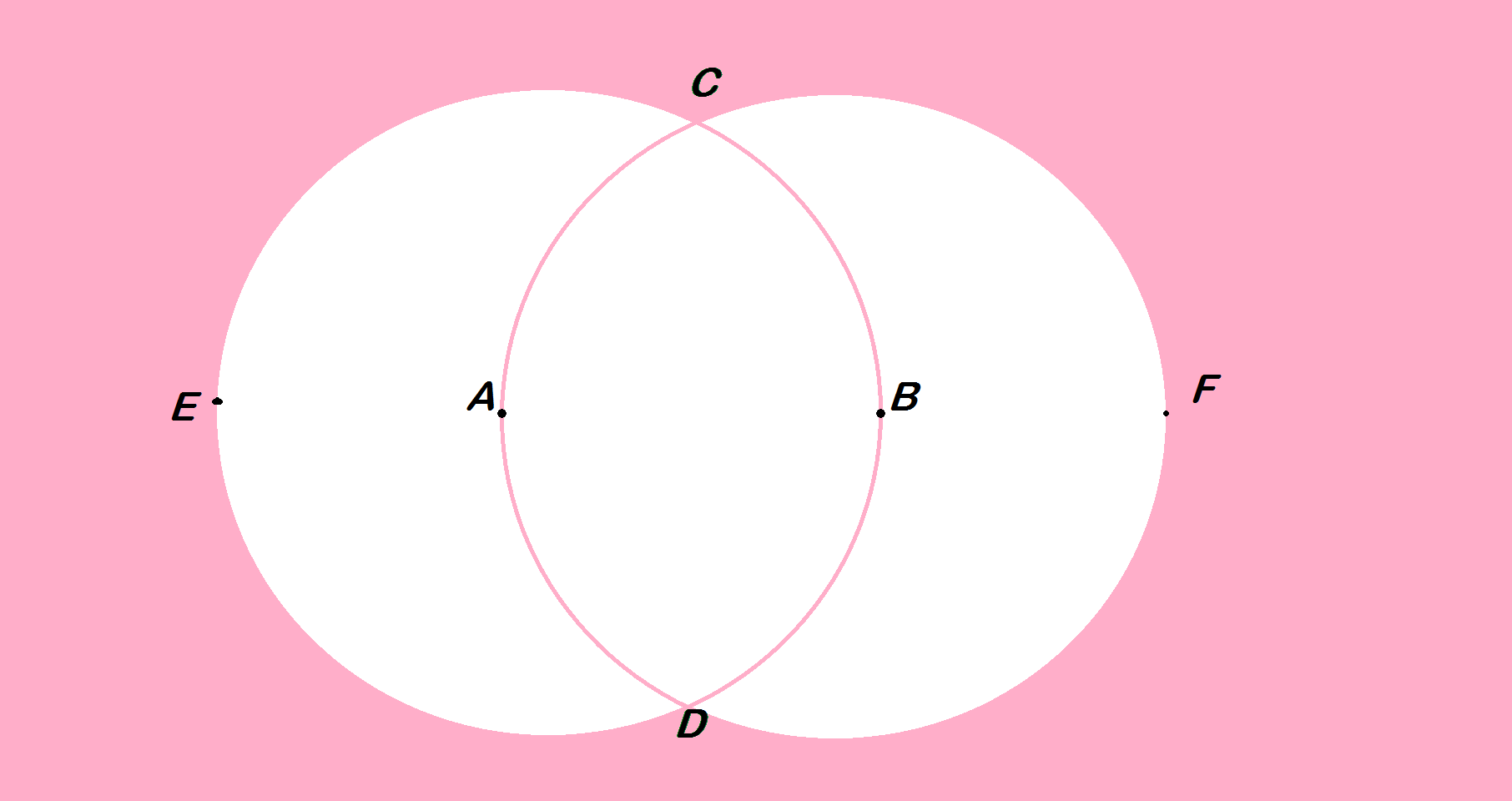# Properties of circle(moving circle). part 3

Geometry Level 2In the above figure, A and B are centres of the circles shown. The circles intersect at C and D.Find $\angle CED$+$\angle CFD$(in degree)

×